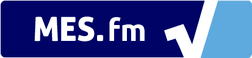We notice that you are using AdBlock. Please whitelist BMI Calculator to help keep this site up and running! :)# BMI Formula

The BMI Formula is displayed in both metric and imperial units of measurement.

A chart of how BMI varies with height and weight can be found here: BMI Chart.

## Metric BMI Formula

BMI =

Mass (Kilograms)

(Height (Meters))2

## Imperial BMI Formula

BMI =

Mass (Pounds)

(Height (Inches))2

× 703*

* The value '703' is more accurately '703.06957964' but higher precision is negligible when calculating BMI.

## BMI Formula Conversion to Imperial Units

The BMI Formula is simply the mass of a person divided by the square of the height and is defined using the metric units of kilograms for mass and meters for height. This means that when using the imperial units of pounds for mass and inches for height, these need to be converted metric unites, which arises the extra factor of 703. This conversion is shown below:

• 1 pound (lb) = 0.453592 kilograms (kg)
• 1 inch (in) = 0.0254 meters (m)

\rightarrow BMI = \frac{Mass (kg)}{[Mass (m)]^2}
= \frac{Mass (lb) * 0.453592 \frac{kg}{in}}{[Height (in) * 0.0254 \frac{m}{in}]^2}
= \frac{Mass(lb)}{[Height (in)]^2} * \frac{0.453592 \frac{kg}{lb}}{0.0254^2 \frac{m^2}{in^2}}
= \frac{Mass(lb)}{[Height (in)]^2} * 703.069 \frac{\frac{kg}{lb}}{\frac{m^2}{in^2}}
\approx \frac{Mass(lb)}{[Height (in)]^2} * 703 \frac{\frac{kg}{lb}}{\frac{m^2}{in^2}}

Please note that the units above for the factor 703 simply convert the imperial units to the metric units and thus the 703 is considered to be a conversion factor. Another note is that the conversion factor is simply rounded up to 703 because the body mass index does not need to have a high level of precision since the BMI bodyweight categories are broad and have at most just 1 decimal place of accuracy.

## Important Notes

The BMI Formula has been widely used because it is very simple and easy to calculate. This allows for the ability to compare many different peoples and even entire countries BMIs. That being said it still has its limitations because it assumes that mass of a person is proportional to the square of the height. This is in fact not always the case and it can be shown that for taller people the mass is actually more proportional to the cube of the height. Thus this pre-defined proportion of mass to the square of the height is only hypothetical and is used to get a general BMI for all body types, genders, and sizes. This simplification makes it easier and quicker to calculate and compare different people and their bodyweights but at the same time it may be inaccurate for some people.

For a full breakdown on the advantages and benefits of the BMI, please go to our BMI Calculator homepage.